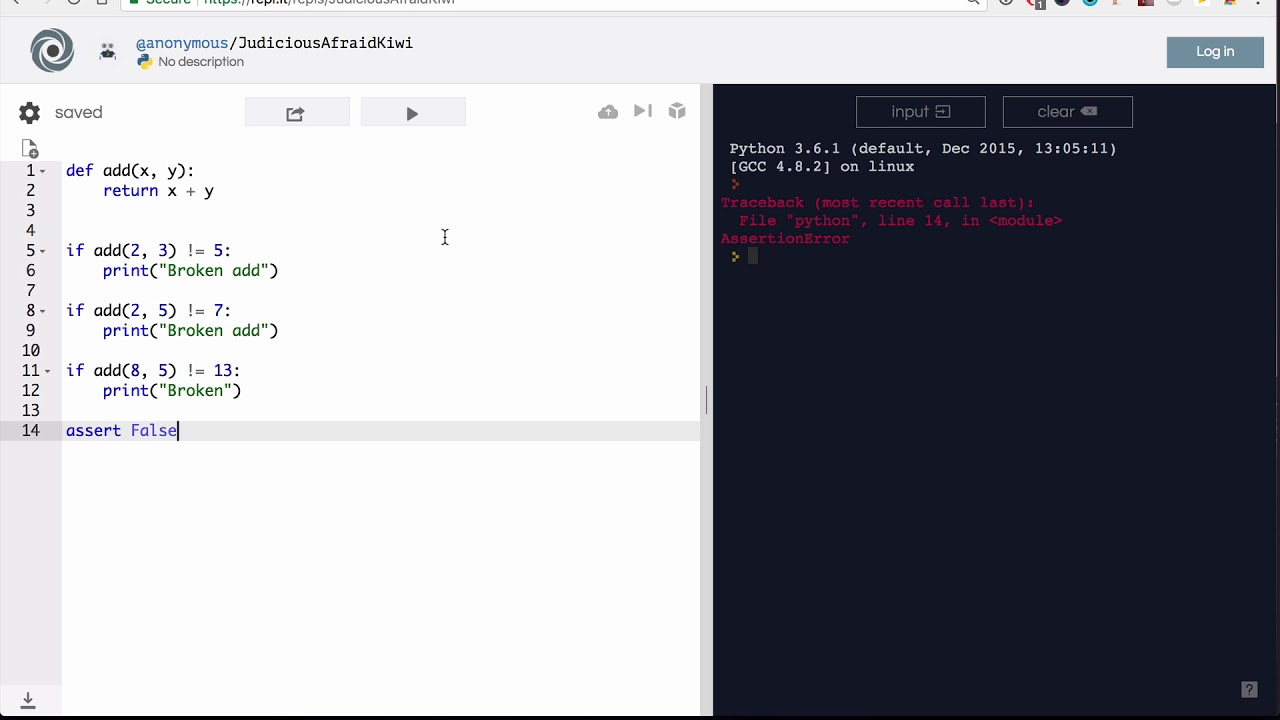### The `assert` statement

The `assert` statement allows us to verify that our code works as expected, in a simple and reliable way. Python interprets `assert` as we'd do: "make sure this current statement is true". Examples:

``````assert 2 + 2 == 4  # make sure "two plus two is equals to four"
assert add(2, 3) == 5  # make sure that "if we invoke add with 2 and 3, the result is 5"
``````

This makes it a really powerful statement and a really useful one to verify our code works. The usual procedure is to focus on the "input" and "output" of your code: what is it receiving ("input") and what is it supposed to return (or produce, the "output"). Assert will let you think about that. Once you've written the assert statement(s) you can focus on writing the actual code.

## An example

Our task is to write a function `is_prime` that, given a number, it'll return `True` or `False` depending on it being prime or not.

#### Step 1: Understand the problem

We'll start by making sure we understand the problem. A great way to do it is by thinking about "examples". I know a couple of prime numbers, for example, I know that:

• `7` is prime
• `11` is prime
• `13` is prime
• `137` is prime
• `4` is NOT prime
• `9` is NOT prime
• `142` is NOT prime

#### Step 2: Write the tests

Now that we've thought the problem, we're going to write the actuall assert statements (tests). This will let us focus on our input and output. We're just translating the previous step, into valid python code.

``````assert is_prime(7) is True
assert is_prime(11) is True
assert is_prime(13) is True
assert is_prime(137) is True
assert is_prime(4) is False
assert is_prime(9) is False
assert is_prime(142) is False
``````

Here you see the regular process of "translation", between your "human" understanding of the problem and the way to express it for Python to process it.

#### Step 3: Write the code

So, we've first written the tests and we haven't even written the code. This is interesting 🤔.